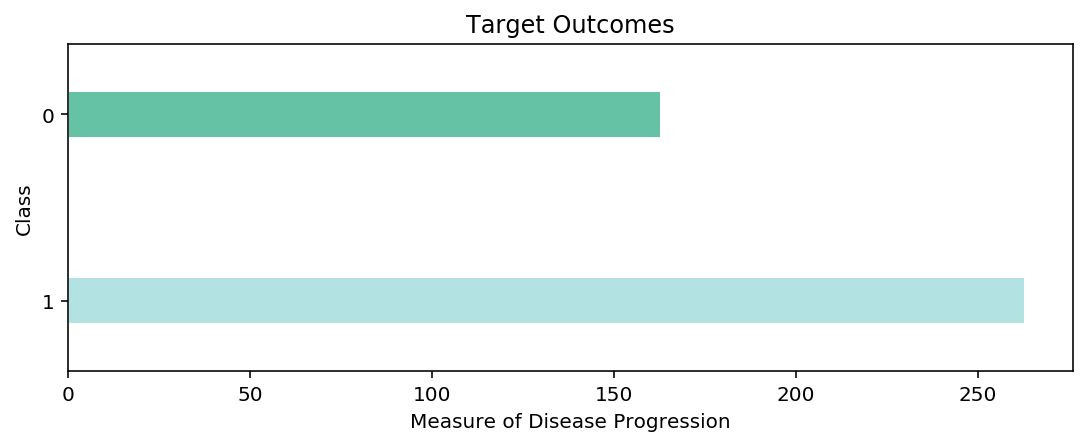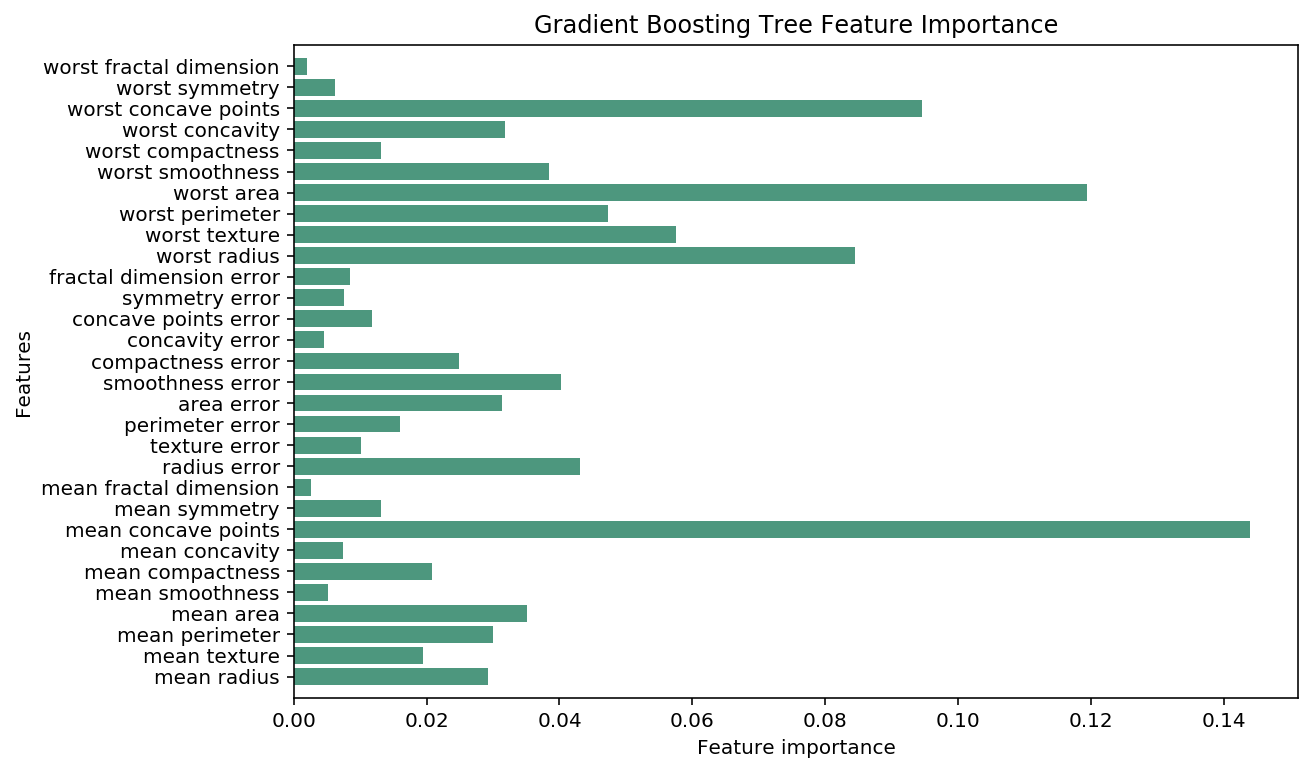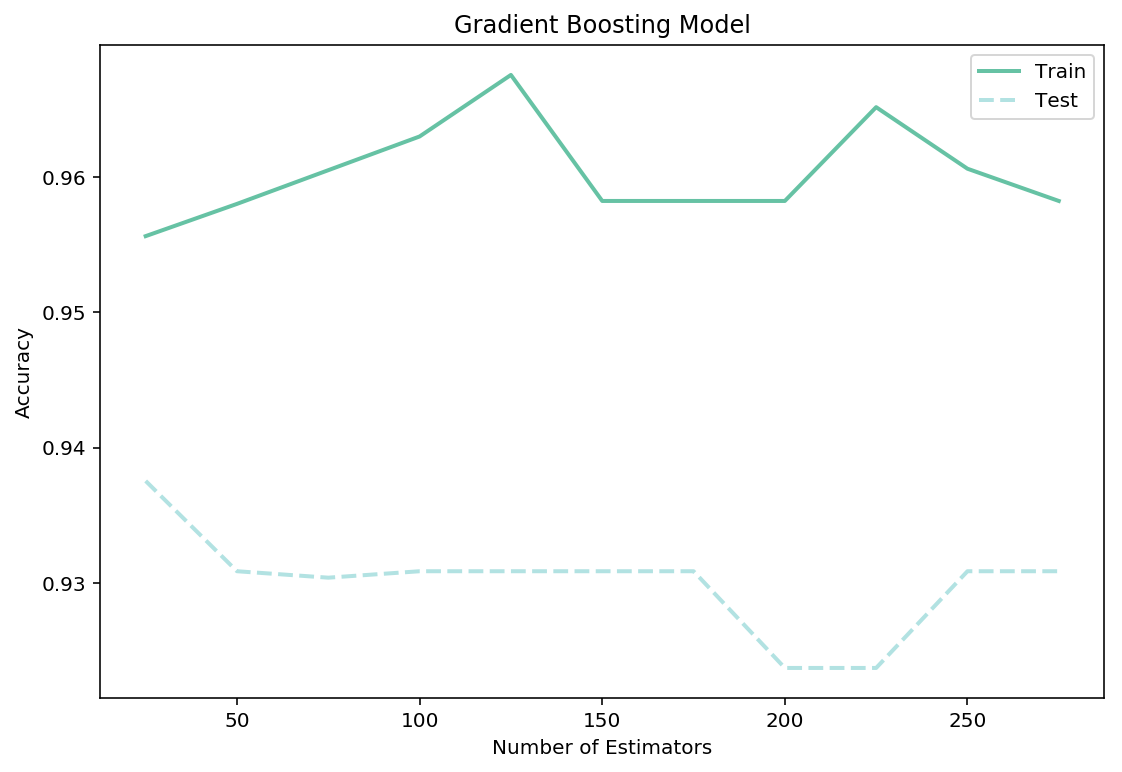15 Sep

## Grandient Boosting Classifier

Gradient Boosting Trees follow the same type of logic as Random Forest Classifiers, instead of using one tree lets build a ensemble. The key differenc here is that instead building all the trees out ina mutually exculsive manner, lets instead build them out sequentially. Where each new tree tries to corret the errors for the last one

In this notebook, I will be looking at the famous breastcancer dataset. This dataset is a multi-class classification problem, where I need to predict the correct target for each observation from a range of possible classes. We will attempt to predict the proper target class using this model, given the feature of each type of class, I often reuse this dataset between my tree-based notebooks. Using the same dataset makes it very easy to compare and contrast the performance of different tree-based models, and keep the trees a reasonable size.

Dataset: Breast Cancer

### Import Preliminaries¶

In :
%matplotlib inline
%config InlineBackend.figure_format='retina'

# Import modules
import matplotlib.pyplot as plt
import matplotlib as mpl
import numpy as np
import pandas as pd
import seaborn
import warnings

from sqlalchemy import create_engine
from sklearn.model_selection import train_test_split, cross_val_score, GridSearchCV

# Set pandas options
pd.set_option('max_columns',1000)
pd.set_option('max_rows',30)
pd.set_option('display.float_format', lambda x: '%.3f' % x)

# Set plotting options
mpl.rcParams['figure.figsize'] = (9.0, 3.0)

# Set warning options
warnings.filterwarnings('ignore');


### Import Data¶

In :
# Import Breast Cancer data
X, y = breast_cancer.data, breast_cancer.target

# Conduct a train-test split on the data
train_x, test_x, train_y, test_y = train_test_split(X,y)

# View the training dataframe

Out:
mean radius mean texture mean perimeter mean area mean smoothness mean compactness mean concavity mean concave points mean symmetry mean fractal dimension radius error texture error perimeter error area error smoothness error compactness error concavity error concave points error symmetry error fractal dimension error worst radius worst texture worst perimeter worst area worst smoothness worst compactness worst concavity worst concave points worst symmetry worst fractal dimension
0 14.540 27.540 96.730 658.800 0.114 0.160 0.164 0.074 0.230 0.071 0.370 1.033 2.879 32.550 0.006 0.042 0.047 0.011 0.019 0.005 17.460 37.130 124.100 943.200 0.168 0.658 0.703 0.171 0.422 0.134
1 25.220 24.910 171.500 1878.000 0.106 0.267 0.334 0.184 0.183 0.068 0.897 1.474 7.382 120.000 0.008 0.057 0.057 0.020 0.011 0.006 30.000 33.620 211.700 2562.000 0.157 0.608 0.648 0.287 0.235 0.105
2 12.460 24.040 83.970 475.900 0.119 0.240 0.227 0.085 0.203 0.082 0.298 1.599 2.039 23.940 0.007 0.072 0.077 0.014 0.018 0.010 15.090 40.680 97.650 711.400 0.185 1.058 1.105 0.221 0.437 0.207
3 12.320 12.390 78.850 464.100 0.103 0.070 0.040 0.037 0.196 0.060 0.236 0.666 1.670 17.430 0.008 0.012 0.017 0.012 0.019 0.002 13.500 15.640 86.970 549.100 0.139 0.127 0.124 0.094 0.283 0.068
4 13.000 21.820 87.500 519.800 0.127 0.193 0.186 0.094 0.235 0.074 0.306 1.002 2.406 24.320 0.006 0.035 0.036 0.012 0.021 0.004 15.490 30.730 106.200 739.300 0.170 0.540 0.539 0.206 0.438 0.107

### Data Overview¶

In :
# Plot a barplot of the target clasees
pd.Series(train_y).value_counts().plot.barh(grid=False, color=['#B2E2E2','#66C2A4'], width=0.25,edgecolor='w')
plt.title('Target Outcomes')
plt.ylabel('Cl
ass')
plt.xlabel('Measure of Disease Progression');### Fit the Model¶

In :
# Fit the intial model
gb_model.fit(train_x, train_y);


### Model Evaluation¶

##### Cross Validation Score¶
In :
# View the cross validation score of the intial model
scores = cross_val_score(gb_model, train_x, train_y, cv=10,
scoring='accuracy')
print(f'Cross Validation Score: {scores.mean():.5f}')

Cross Validation Score: 0.96050

##### Confustion Matrix¶
In :
# Training confusion matrix
from sklearn.metrics import confusion_matrix
cmatrix = pd.DataFrame(confusion_matrix(train_y, gb_model.predict(train_x)))
cmatrix.index.name = 'class'

cmatrix['result'] = 'actual'
cmatrix.set_index('result', append=True, inplace=True)
cmatrix = cmatrix.reorder_levels(['result', 'class'])
cmatrix = cmatrix.stack()
cmatrix = pd.DataFrame(cmatrix)
cmatrix.columns = ['prediction']
cmatrix.unstack()

Out:
prediction
0 1
result class
actual 0 163 0
1 0 263
##### Feature Importance¶
In :
# Plot ensemble's feature importance
mpl.rcParams['figure.figsize'] = (9.0, 6.0)
n_features = breast_cancer.data.shape
plt.barh(range(n_features), gb_model.feature_importances_, align='center', color='#4D977E')
plt.yticks(np.arange(n_features), breast_cancer.feature_names)
plt.xlabel("Feature importance")
plt.ylabel("Features")
plt.ylim(-1, n_features);##### Parameter Tuning¶
In :
# Define paraameter range and score lists
n_estimators_range = np.arange(0,300, 25)[1:]
train_score = []
test_score = []

# Train a knn_model for every neighbour value in our list
for i in n_estimators_range:
train_score.append(cross_val_score(gb_model, train_x, train_y, cv=10, scoring='accuracy').mean())
test_score.append(cross_val_score(gb_model, test_x, test_y, cv=10, scoring='accuracy').mean())

# Plot our results
mpl.rcParams['figure.figsize'] = (9.0, 6.0)
plt.plot(n_estimators_range,train_score,label="Train",linewidth=2, color='#66C2A4')
plt.plot(n_estimators_range,test_score,label="Test", linewidth=2,linestyle='--', color='#B2E2E2')
plt.legend()
plt.xlabel('Number of Estimators')
plt.ylabel('Accuracy');##### Grid Searching Turnning¶
In :
# Set up parameter grid
grid = {'learning_rate':np.arange(0.1,1.0,0.2),
'n_estimators':np.arange(0,100, 25)[1:],
'max_depth':list(range(2,30,4)),
'max_features': list(range(2,30,2)),
'max_leaf_nodes':[5,10,25,50,75,100]}

# Conduct gird search
grid_search = GridSearchCV(estimator=rf_model, param_grid=grid,
scoring='accuracy', n_jobs=-1, refit=True, cv=10,
return_train_score=True)
# Fit model
grid_search.fit(train_x,train_y);

---------------------------------------------------------------------------
NameError                                 Traceback (most recent call last)
<ipython-input-12-df90f3092c03> in <module>()
7
8 # Conduct gird search
----> 9 grid_search = GridSearchCV(estimator=rf_model, param_grid=grid,
10                      scoring='accuracy', n_jobs=-1, refit=True, cv=10,
11                      return_train_score=True)

NameError: name 'rf_model' is not defined
In [ ]:
print('Accuracy of best parameters: %.5f'%grid_search.best_score_)
print('Best parameters: %s' %grid_search.best_params_)


### Final Model¶

In [ ]:
# Fit the final model
max_depth=2, max_features=10,
max_leaf_nodes=50, n_estimators=50)
gd_model.fit(train_x, train_y)

# View the cross validation score of the intial model
scores = cross_val_score(gb_model, train_x, train_y, cv=10,
scoring='accuracy')
print(f'Cross Validation Score: {scores.mean():.5f}')

##### Confustion Matrix¶
In [ ]:
# Training confusion matix
from sklearn.metrics import confusion_matrix
cmatrix = pd.DataFrame(confusion_matrix(train_y, gb_model.predict(train_x)))
cmatrix.index.name = 'class'

cmatrix['result'] = 'actual'
cmatrix.set_index('result', append=True, inplace=True)
cmatrix = cmatrix.reorder_levels(['result', 'class'])
cmatrix = cmatrix.stack()
cmatrix = pd.DataFrame(cmatrix)
cmatrix.columns = ['prediction']
cmatrix.unstack()

##### Feature Importance¶
In [ ]:
# Plot ensembles's feature importance
n_features = breast_cancer.data.shape
plt.barh(range(n_features),gb_model.feature_importances_, align='center', color='#4D977E')
plt.yticks(np.arange(n_features), breast_cancer.feature_names)
plt.xlabel("Feature importance")
plt.ylabel("Features")
plt.ylim(-1, n_features);


### Predict Results¶

In [ ]:
# Predict the results from our test data